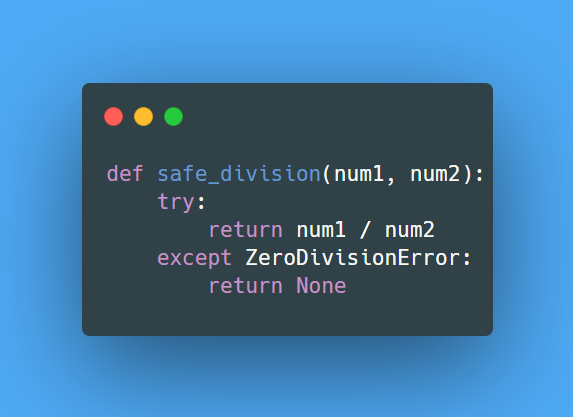Lesson 1 of 0
In Progress

# Division Safety Check

##### Kedeisha June 16, 2023

Write a Python function that accepts two numbers and performs division operation. The function should return the result if the operation is successful, else it should return `None`. The function should handle `ZeroDivisionError` exception.

#### Input Data:

• Your function should accept two parameters:
1. `numerator`: A number representing the numerator in the division operation.
2. `denominator`: A number representing the denominator in the division operation.

#### Example:

1. If the input is `numerator = 10` and `denominator = 2`, then the output should be `5`.
2. If the input is `numerator = 10` and `denominator = 0`, then the output should be `None`.Explanation of Solution: The function `safe_division` attempts to divide the first argument by the second. If the second argument is zero, a `ZeroDivisionError` will be raised, and the function will return `None`. If no exceptions are raised, the function will return the result of the division operation.